Next: Discussion Up: Quantitative Comparisons with Previous: Predicted Orientation Contrast

## Second Order Effect

The theory not only explains the first order effects which are dominant in angle range of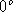to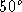, but also accounts for the second order effects which can be seen into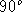range, where the sign of the effects is reversed.

If one includes the second order effect, it can be considered as an effective cross-orientation (90 degrees) interaction of range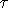and the strength of decorrelation feedback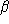. For the orientation adaptation, the Equation 11 is now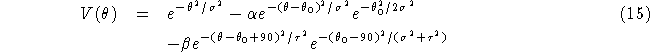For the orientation contrast, the Equation 13 is now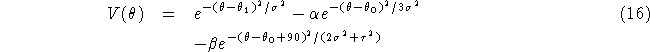The comparison of the theoretical predictions with the experimental data is shown in Figure 6. The parameters used are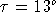for both effects and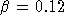and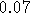for the orientation adaptation and orientation contrast respectively. Note that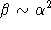for both effects, andis much smaller than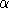and thus for second order effect.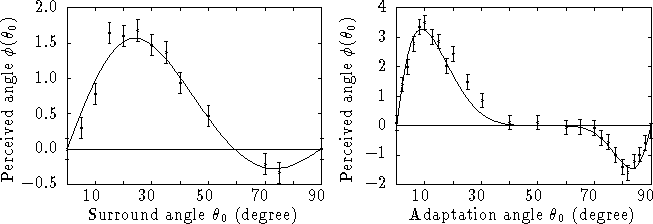Figure 6: Quantitative comparison of the theoretical predictions with the experimental data of orientation contrast (left) and orientation adaptation (right). This figure includes second order effec.Next: Discussion Up: Quantitative Comparisons with Previous: Predicted Orientation Contrast